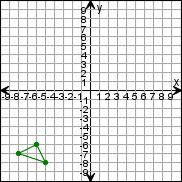Create a new printableGeometry
Math Worksheets

Sample - Click above to make a new math worksheet (PDF).
 Name _____________________________Date ___________________
Coordinate Plane and Transformations
Write the coordinates for each point.
 1 * This is a pre-made sheet.Use the link at the top of the page for a printable page.
2.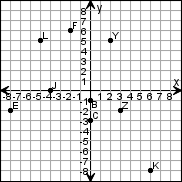J _______

 Z _______

 B _______

 L _______

 F _______

Name the quadrant or on which axis the point lies.
3.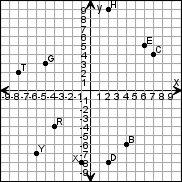G _______

 C _______

 B _______

 X _______

 E _______
4.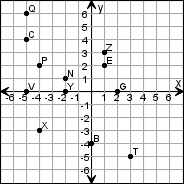E _______

 P _______

 Q _______

 N _______

 B _______

Complete the function table and then graph the function.
5.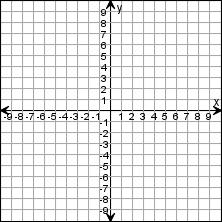y = 5 + 4x
 x y -2 -1 0 1
6.y = 4x + 1
 x y -2 -1 0 1

 Key #2
 aKey #2
Make a function table for the line. Write an equation for this function.
7.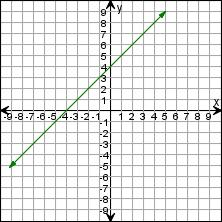x y
8.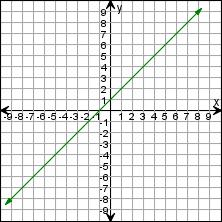x y

Draw the triangle after the transformations.
 9 Translate the triangle down 3 units, then 2 units right.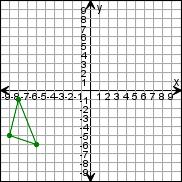10 Translate the triangle down 9 units, then 5 units left.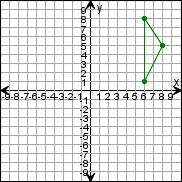11 Translate the triangle 1 unit right.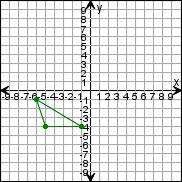Draw the triangle after the transformations.
 12 Reflect the triangle over the x-axis.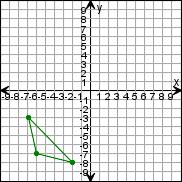13 Reflect the triangle over the y-axis.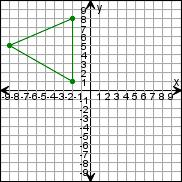14 Reflect the triangle over the y-axis.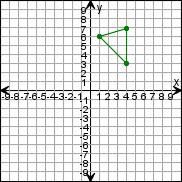Key #2
 aKey #2
Draw the triangle after the transformations.
 15 Rotate the triangle 270clockwise around (0,0).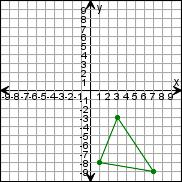16 Rotate the triangle 180clockwise around (0,0).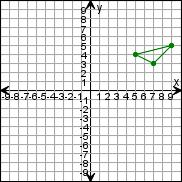17 Rotate the triangle 180clockwise around (0,0).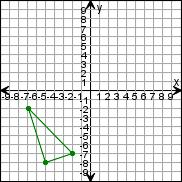Draw the triangle after the transformations.
 18 Translate the triangle up 8 units, then 7 units left.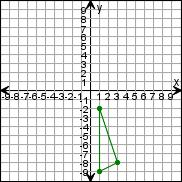19 Rotate the triangle 180clockwise around (0,0).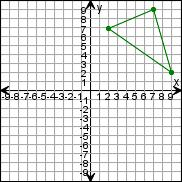20 Reflect the triangle over the x-axis.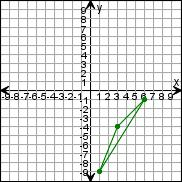Draw the triangle after the transformations.
 21 Translate the triangle 1 unit right. Then rotate the new triangle 270clockwise around (0,0).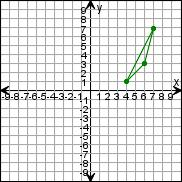22 Translate the triangle up 4 units, then 3 units right. Then reflect the new triangle over the x-axis.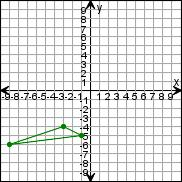23 Translate the triangle left 1 unit, then 1 unit up. Then rotate the new triangle 270clockwise around (0,0).